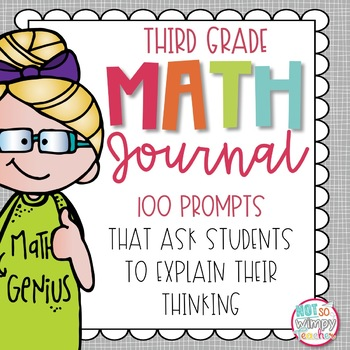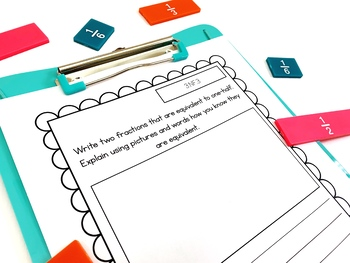Total:
\$0.00# Word Problem Math Journal for Third Grade *Editable*3rd
Subjects
Standards
Resource Type
Formats Included
• Zip
•Google Apps™
Pages
250 pagesThe Teacher-Author indicated this resource includes assets from Google Workspace (e.g. docs, slides, etc.).

### Description

This journal is so much more than a collection of word problems. These journal prompts ask students to defend their answers, list the steps they took to solve the problem, make their own problems, draw pictures, etc. Writing about their mathematical thinking will help students to prepare for standardized testing and show a true understanding of the math concepts rather than just test if the student gets the right answer.

**************************************

HUGE UPDATE:

This resource has been updated to include FOUR formats for each prompt, an answer key and editable slides!

Formats:

1. Full page prompts:

They can be printed on a single sheet of paper and used as an assessment or homework assignment. They can also be glued into a spiral or composition notebook.

2. Strips:

One page includes several copies of the same prompt. This is a paper saver! Students can glue the strip to the top of a sheet of paper or into their math notebook. It's perfect for centers, morning work, fast finisher work, etc.

3. PowerPoint:

This is a no prep option! Just display the desired slide on your board and students can respond to the question in their notebook. It is perfect for bell work, math warm up or an exit ticket at the end of a lesson.

These slides make it easy to assign a prompt in Google Classroom! Students can respond to the prompt directly on the slide.

*******************************

Every single 3rd grade common core math standard is covered!

There are many different ways you could use this product:

Independent center work during math

Group assignment during math

Morning bell work

Math warm-up

Homework

Fast-finisher Activity

Assessments

Exit Tickets

Unit includes:

Suggested Uses

Preparation Instructions

Suggestions for implementing and teaching students to write about math

Student journal cover

Check-off sheet to keep track of prompts used

Math Journal Sentence Starters

100 full page journal prompts*

100 journal prompt strips*

100 journal prompt PowerPoint slides*

100 journal prompts in Google Slides*

Editable Full Page Template

Editable PowerPoint Template

*The same prompts are in each file. They are just included in three different formats.

To see how I use my math journals in my classroom check my blog post: Write about Math

I also have this product for other grade levels and they will be updates SOON!

You might also be interested in:

Math Interactive Notebooks

Total Pages
250 pages
Included
Teaching Duration
1 Year
Report this Resource to TpT
Reported resources will be reviewed by our team. Report this resource to let us know if this resource violates TpT’s content guidelines.

### Standards

to see state-specific standards (only available in the US).
Interpret products of whole numbers, e.g., interpret 5 × 7 as the total number of objects in 5 groups of 7 objects each. For example, describe a context in which a total number of objects can be expressed as 5 × 7.
Interpret whole-number quotients of whole numbers, e.g., interpret 56 ÷ 8 as the number of objects in each share when 56 objects are partitioned equally into 8 shares, or as a number of shares when 56 objects are partitioned into equal shares of 8 objects each. For example, describe a context in which a number of shares or a number of groups can be expressed as 56 ÷ 8.
Use multiplication and division within 100 to solve word problems in situations involving equal groups, arrays, and measurement quantities, e.g., by using drawings and equations with a symbol for the unknown number to represent the problem.
Determine the unknown whole number in a multiplication or division equation relating three whole numbers. For example, determine the unknown number that makes the equation true in each of the equations 8 × ? = 48, 5 = __ ÷ 3, 6 × 6 = ?.
Apply properties of operations as strategies to multiply and divide. Examples: If 6 × 4 = 24 is known, then 4 × 6 = 24 is also known. (Commutative property of multiplication.) 3 × 5 × 2 can be found by 3 × 5 = 15, then 15 × 2 = 30, or by 5 × 2 = 10, then 3 × 10 = 30. (Associative property of multiplication.) Knowing that 8 × 5 = 40 and 8 × 2 = 16, one can find 8 × 7 as 8 × (5 + 2) = (8 × 5) + (8 × 2) = 40 + 16 = 56. (Distributive property.)